Question

# use implicit differentiation to find the slope of the tangent line to the curve (y)/(x-7y)=x^6+5 at the point (1,6/43)

use implicit differentiation to find the slope of the tangent line to the curve (y)/(x-7y)=x^6+5 at the point (1,6/43)

when you cross multiply how do you get 36y?
THANK YOU !
when you cross multiply how do you get 36y?
I would cross-multiply, then simplify to avoid using the quotient rule to get

36y = x^7 + 5x - 7x^6y
36dy/dx = 7x^6 + 5 -7x^6dy/dx - y(42x^5)
dy/dx(36 + 7x^6) = 7x^6 + 5x - 42yx^5
dy/dx = (7x^6 + 5x - 42yx^5)/(36+7x^6)

sub in x=1 and y = 6/43)

I will leave the arithmetic for you

#### Earn Coins

Coins can be redeemed for fabulous gifts.

Similar Homework Help Questions
• ### (1 point) Use implicit differentiation to find the slope of the tangent line to the curve...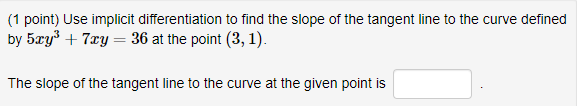(1 point) Use implicit differentiation to find the slope of the tangent line to the curve defined by 5xy + 7xy = 36 at the point (3,1). The slope of the tangent line to the curve at the given point is

• ### Use implicit differentiation to find the slope of the tangent line to the curve 5x^3 y^2...

Use implicit differentiation to find the slope of the tangent line to the curve 5x^3 y^2 - 4x^2 y = 1at the point (1,1) m=

• ### (1 point) Use implicit differentiation to find an equation of the tangent line to the curve...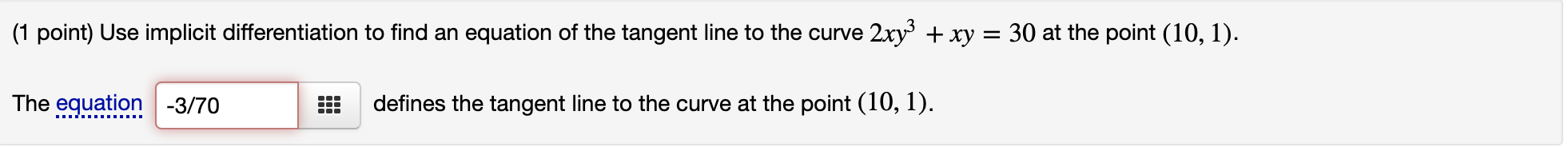(1 point) Use implicit differentiation to find an equation of the tangent line to the curve 2xy3+xy=302xy3+xy=30 at the point (10,1)(10,1). (1 point) Use implicit differentiation to find an equation of the tangent line to the curve 2xy3 + xy = 30 at the point (10, 1). The equation -3/70 defines the tangent line to the curve at the point (10, 1).

• ### Consider the curve y = x ? x3. Find the slope of the tangent line at the point (1,6).

Consider the curve y = x − x3. Find the slope of the tangent line at the point (1,6).

• ### Use implicit differentiation to find the equation of the tangent line to the curve xy^3+xy=5 at the point (4,1)

Use implicit differentiation to find the equation of the tangent line to the curve xy^3+xy=5 at the point (4,1) . The equation of this tangent line can be written in the form y = mx+b where m is:? and where b is:?

• ### Find the equation of the tangent line to the curve at the given point using implicit...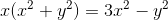Find the equation of the tangent line to the curve at the given point using implicit differentiation. Remember: equation of a line can be found by y-y1=m(x-x1) where m is the slope of the line and (x1,y1) is any point on the line. Curve: at (1,1)

• ### Use implicit differentiation to find the equation of the tangent line to the curve xy3+xy=14 at the point (7,1)

Use implicit differentiation to find the equation of the tangent line to the curve xy3+xy=14 at the point (7,1) . The equation of this tangent line can be written in the form y=mx+bi dont seem to no how to find m or b

• ### Use implicit differentiation to find the equation of the tangent line to the curve xy^3+2xy=9 at the point (31)

Use implicit differentiation to find the equation of the tangent line to the curve xy^3+2xy=9 at the point (31). The equation of this tangent line can be written in the form y=mx+b where m is

• ### Use implicit differentiation to find the equation of the tangent line at the given point. z?...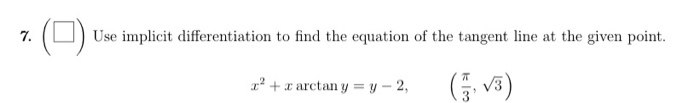Use implicit differentiation to find the equation of the tangent line at the given point. z? + x arctan y = y -2,

• ### Use implicit differentiation to find an equation of the tangent line to the graph of the...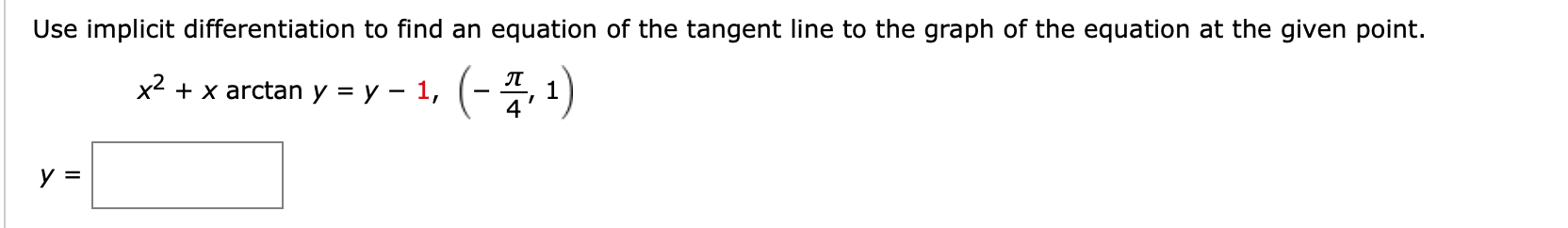Use implicit differentiation to find an equation of the tangent line to the graph of the equation at the given point. x2 + x arctan y = y -